# Photos from Crude Sketches: NVIDIA's GauGAN Explained VisuallyMachine Learning Engineer

Last month at NVIDIA GTC 2019, NVIDIA unveiled a new app that turns simple blobs of color drawn by a user into dazzling photorealistic paintings.

The app builds off the deep learning–based technology of generative adversarial networks (GAN). In a pun inspired by painter Paul Gauguin, NVIDIA has named it GauGAN. Underlying GauGAN’s functionality is a new algorithm called SPADE.

In this article I’ll be explaining how this feat of engineering works from the ground up. To engage as many interested readers as possible, I’ll assume little more than a basic understanding of convolutional neural networks. Since SPADE is a generative adversarial network, I’ll be covering them in some detail. But if you’re already familiar, you can skip to Image-to-image translation below.

## Generating images

Let’s start with a helpful distinction: most current applications in deep learning use a discriminative type of neural network (a discriminator), while SPADE is a generative neural network (a generator).

### Discriminators

A discriminator performs classification of its inputs. For example an image classifier is a discriminator that takes in an image and chooses one appropriate class label such as “dog”, “car” or “traffic light” that describes the image as a whole. Its output is typically represented as a vector of numbers $\vec{v}$ where $\vec{v}_i$ is a number from 0 to 1 representing the network’s confidence that the image belongs to $i$th class.

A discriminator can also make an entire image of classifications. It might classify each pixel in an image as belonging to “people” or “cars” (called “semantic segmentation”).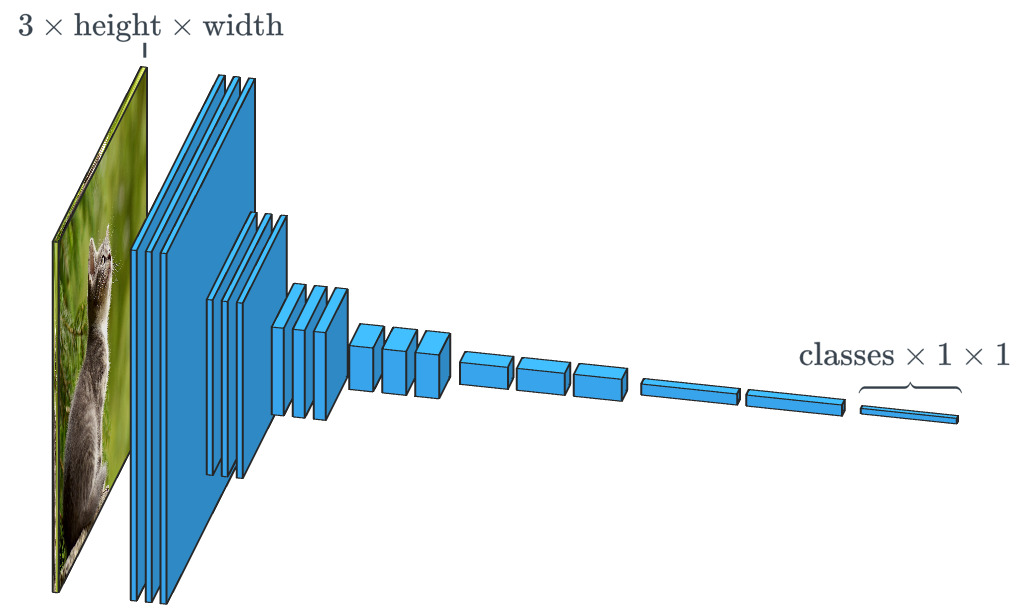An image classifier takes an image with 3 channels (red, green and blue) and maps it to a vector of confidences in each possible class the image might represent.

Since the relationship between an image and its class is very complicated, neural networks feed it through a stack of many layers, each of which processes it a little and passes its output onto the next.

### Generators

A generative network like SPADE is given a dataset and aims to create new, original data that look like they belong to that dataset. The data could be sounds, language or other kinds of data, but we’ll focus on images. In a common case, the input to such a network is just a vector of random numbers, with each possible input producing a different image.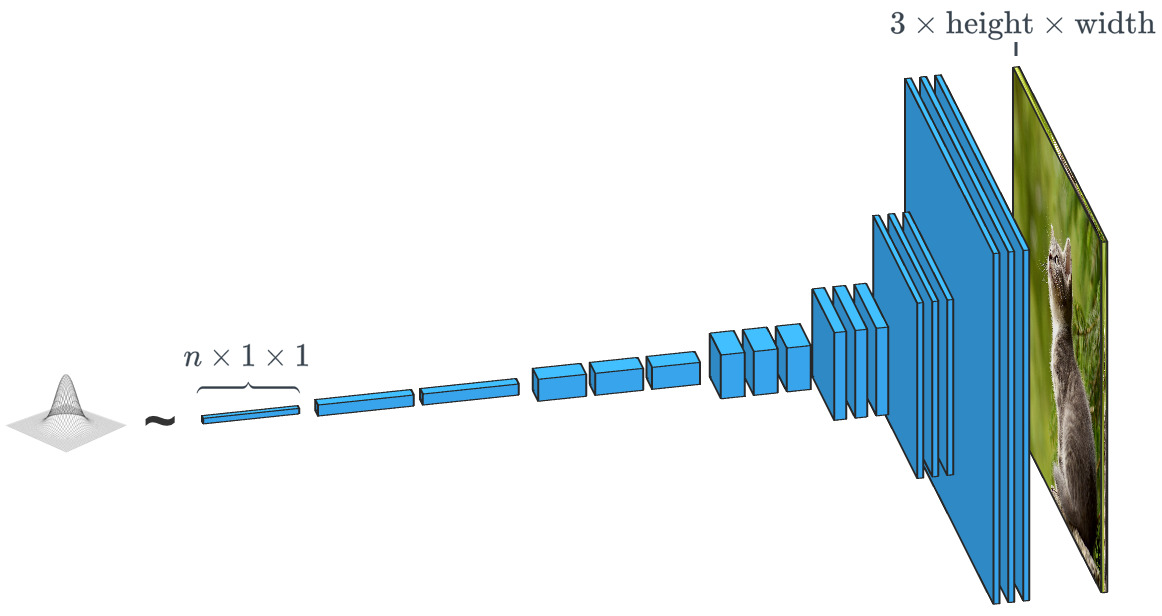A generator based on a random input vector is close to the opposite of an image classifier. In "class-conditional" generators, the input vector is actually a class vector.

As we’ve seen, SPADE uses more than a random vector. It’s guided by a drawing called a segmentation map that tells it what kind of stuff to put where. SPADE does the opposite of the semantic segmentation mentioned above. In general, a discriminative task mapping one type of data to another has the analogous generative task of going the other way.

Modern generators and discriminators typically use convolutions to process their data. For a refresher on convolutional neural networks (CNNs), see Ujjwal Karn, or for more detail Andrej Karpathy’s material.

One important difference between an image classifier and a generator is how they change the size of an image as they work with it. An image classifier needs to scale an image down until it loses all spatial information and only the classes remain. It can do so with pooling layers or by using strided convolutions that skip pixels. A generator builds an image up by using a sort of convolution in reverse called a transposed convolution (or misleadingly a “deconvolution”).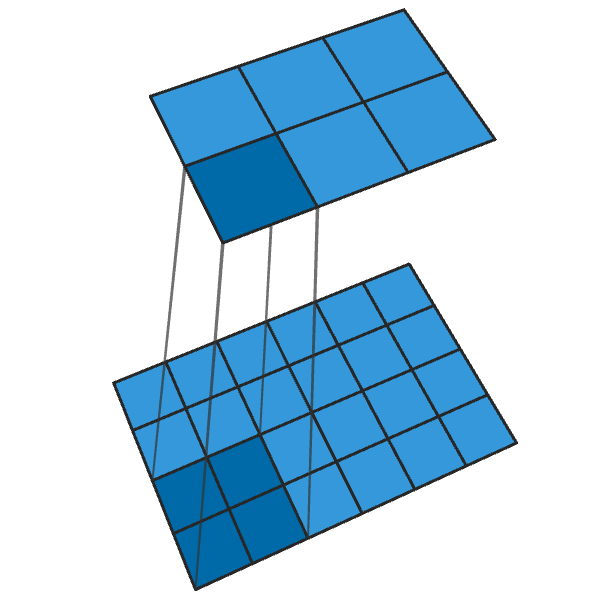A normal 2x2 convolution with a stride of 2 turns every 2x2 block into a single point, reducing the output dimensions by 1/2.

## Training a generator

In theory a convolutional neural network can generate images this way. But how do we train it? That is, given a dataset of images, how do we adjust the parameters of a generator like SPADE so that it produces new images that look like they belong to the dataset?

Compare with image classifiers, where each image in the dataset has a correct class label. Knowing the network’s prediction vector and the correct class, we can use the backpropagation algorithm to determine how the network parameters can be updated to increase its confidence in the correct class and decrease its confidence in the rest.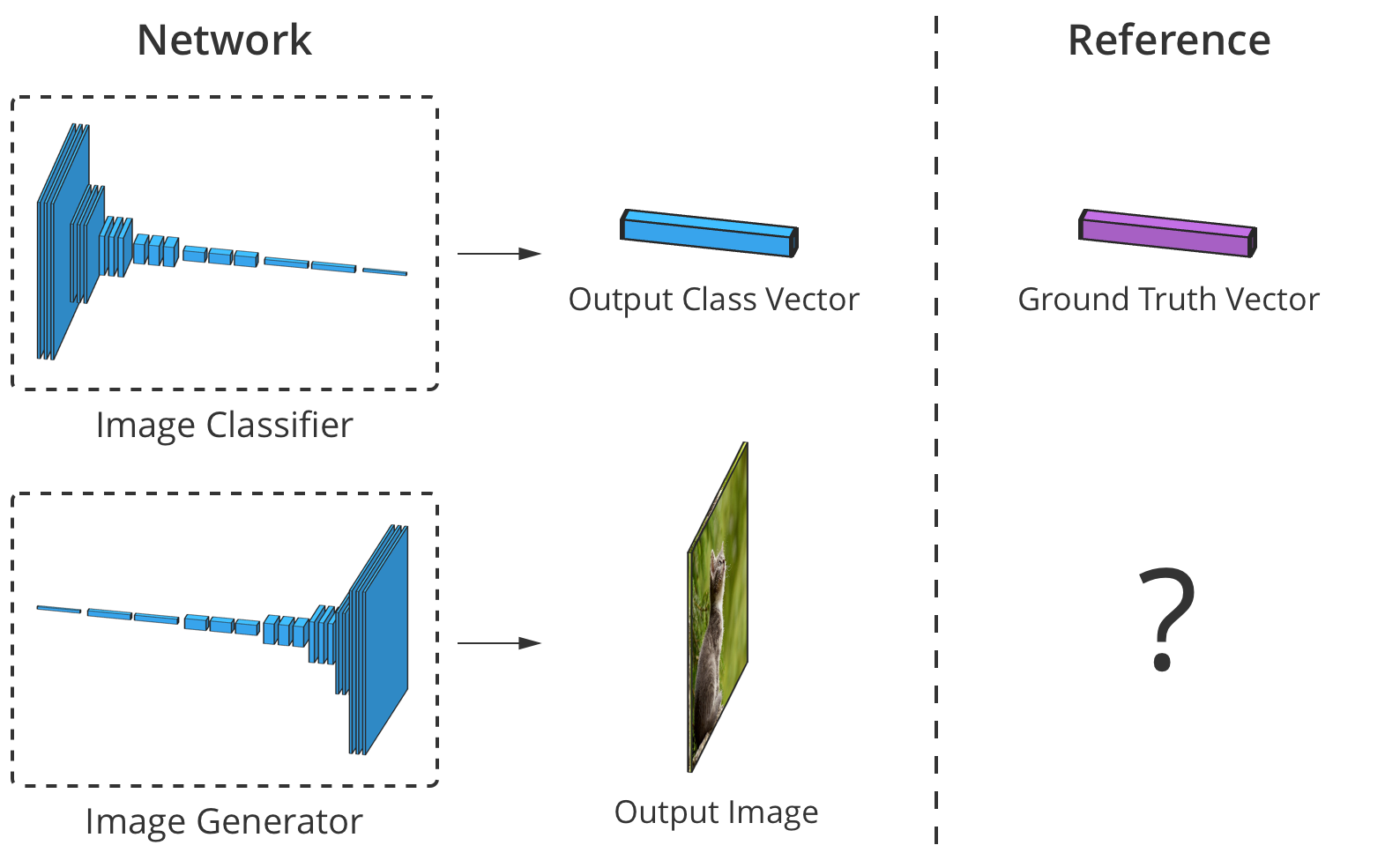An image classifier can be judged by comparing its output to the correct class vector, element-wise. But for generators there's no "correct" output image.

But when a generator produces an image, there are no “correct” values for each pixel. In theory any image that looks plausibly like it belongs to the dataset is valid, even if its pixel values are very different from those images.

So how do we tell the generator at which pixels it should change its output and in what way to produce more realistic images (an “error signal”)? Researchers have pondered this question a great deal and it’s actually quite difficult. Most ideas, such as computing some kind of average “distance” to the real images, produce images that are blurry and of low quality.

Ideally, we would measure how realistic generated images are by using a high-level concept like “How hard is this image to distinguish from the real ones?”…

## Generative adversarial networks

That very goal was achieved by Goodfellow et al., 2014. The idea is to generate images using two neural networks: a generator, and an image classifier (a discriminator). The discriminator is tasked with distinguishing the generator’s output images from real images from the dataset (its classes are “fake” and “real”), while the generator’s job is to fool the discriminator by producing images that look like those in the dataset. One could say the generator and discriminator are adversaries in this setup. Hence its name: a generative adversarial network (GAN).

How does this help? Now we can use an error signal based entirely on the discriminator’s prediction: a single value between 0 (“fake”) to 1 (“real”). Because the discriminator is a neural network, we can backpropagate errors to its input and through to the generator. This tells the generator where and how it can adjust its images to better fool the discriminator (i.e. increase the realism of its images).

As the discriminator learns to detect fake images, it gives the generator better and better feedback on how it can improve the images it produces. In this way the discriminator learns a loss function for the generator.

## Get notified of new posts by email:

### The Goodfellow GAN

The original GAN follows the diagram above. Its discriminator $D$ takes an image $\vec{x}$ and outputs a single value $D(\vec{x})$ between 0 and 1, representing its confidence that $\vec{x}$ is from the dataset rather than a fake image from the generator. Its generator $G$ takes a random vector of normally-distributed numbers $\vec{z}$ and outputs an image $G(\vec{z})$ with which it hopes to fool the discriminator (make $D(G(\vec{z}))$ large).

One issue we haven’t discussed is how GANs are trained and what loss function they use to measure their performance. In general, the loss function should go up as the discriminator learns and down as the generator learns. The loss function of the original GAN used two terms representing 1) a measure of how often the discriminator classifies real images as real and 2) how well it detects fake images:

$$\begin{equation*} \mathcal{L}_\text{GAN}(D, G) = \underbrace{E_{\vec{x} \sim p_\text{data}}[\log D(\vec{x})]}_{\text{accuracy on real images}} + \underbrace{E_{\vec{z} \sim \mathcal{N}}[\log (1 - D(G(\vec{z}))]}_{\text{accuracy on fakes}} \end{equation*}$$

The discriminator $D$ outputs its confidence that an image is real. So it makes sense that $\log D(\vec{x})$ goes up when $D$ thinks $\vec{x}$ is real. And as $D$ gets better at detecting a fake images $G(\vec{z})$, then $D(G(\vec{z}))$ will be close to $0$, so $1 - D(G(\vec{z}))$ will increase as well.

In practice we estimate the accuracy by using batches. We take many (but not all) real images $\vec{x}$ and many random vectors $\vec{z}$ and average the numbers above. Then we backpropagate errors and update adjust $D$’s parameters slightly to increase $\mathcal{L}_{GAN}$ and $G$’s parameters to decrease it.

Over time this leads to some interesting results: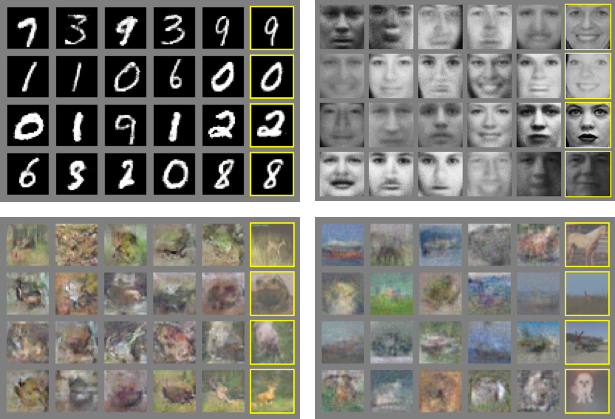The Goodfellow GAN mimicking the MNIST, TFD and CIFAR-10 datasets. Outlined images are the closest in the dataset to the adjacent fakes. Image from paper

This was fantastic for its time, a mere 4.5 years ago. Fortunately, as SPADE and others will show, machine learning continues to make rapid progress.

### Trouble with training

Generative adversarial networks are somewhat notorious for their difficult and unstable training. One problem is that if the generator gets too far ahead of the discriminator during training then it can begin to produce little variety of images — the few that best fool the discriminator. In fact, eventually the generator will output the single optimal image unless the discriminator is trained to keep it in check. This problem is called mode collapse.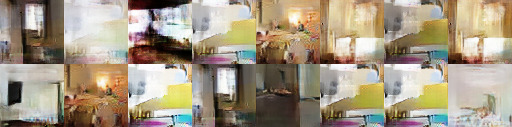Mode collapse of a GAN similar to Goodfellow's. Notice that many of these bedroom images look very similar to each other. Source

Another issue is that when the generator effectively fools the discriminator ($D(G(\vec{z}))$ is large), $\mathcal{L}_\text{GAN}$ provides very small gradients, so $G(\vec{z})$ may not be pushed sufficiently toward the true data distribution $p_\text{data}$ where it would look more realistic.

Research efforts to solve these problems have proceeded largely in the direction of redesigning the loss function $\mathcal{L}_\text{GAN}$. One such simple change proposed by Xudong Mao et al., 2016 is to replace the loss function with a pair of simple least squares-based functions $V_\text{LSGAN}$. This results in more stable training, higher-quality images and less mode collapse and non-vanishing gradients.

Another problem researchers ran into is the difficulty of producing high-resolution images due in part to the fact that a more detailed image gives the discriminator more information to detect fake images. State-of-the-art GANs now start training the network at low resolutions and gradually add more layers until the desired resolution is reached.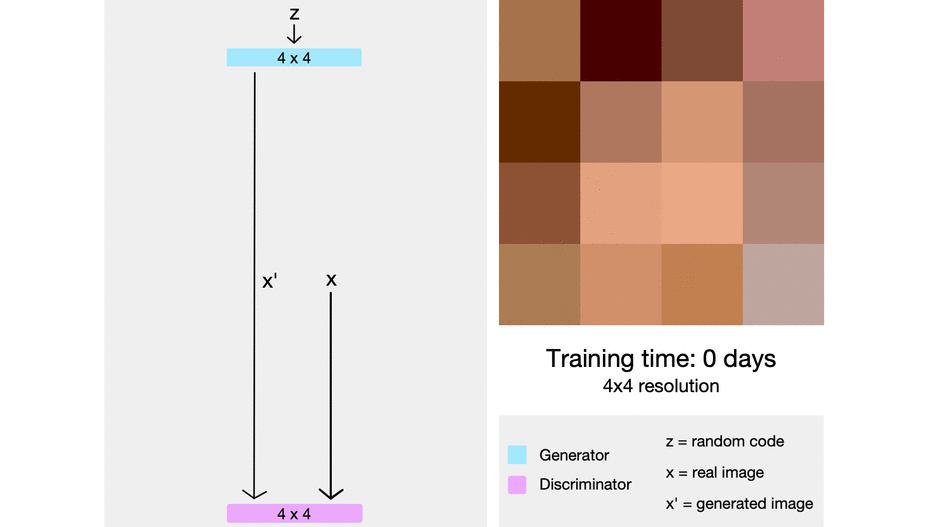Progressively adding higher-resolution layers during training of a GAN substantially improves training stability, speed and the resultant image quality. Image from Sarah Wolf's article on this network, called ProGAN.

## Image-to-image translation

So far we’ve talked about how to generate images from random inputs. But SPADE doesn’t just use random inputs. It uses an image called a segmentation map that assigns to each pixel a class of stuff (e.g. grass, tree, water, rock, sky). And from that image it produces what looks like a photo conforming to that map. This is a type of image-to-image translation.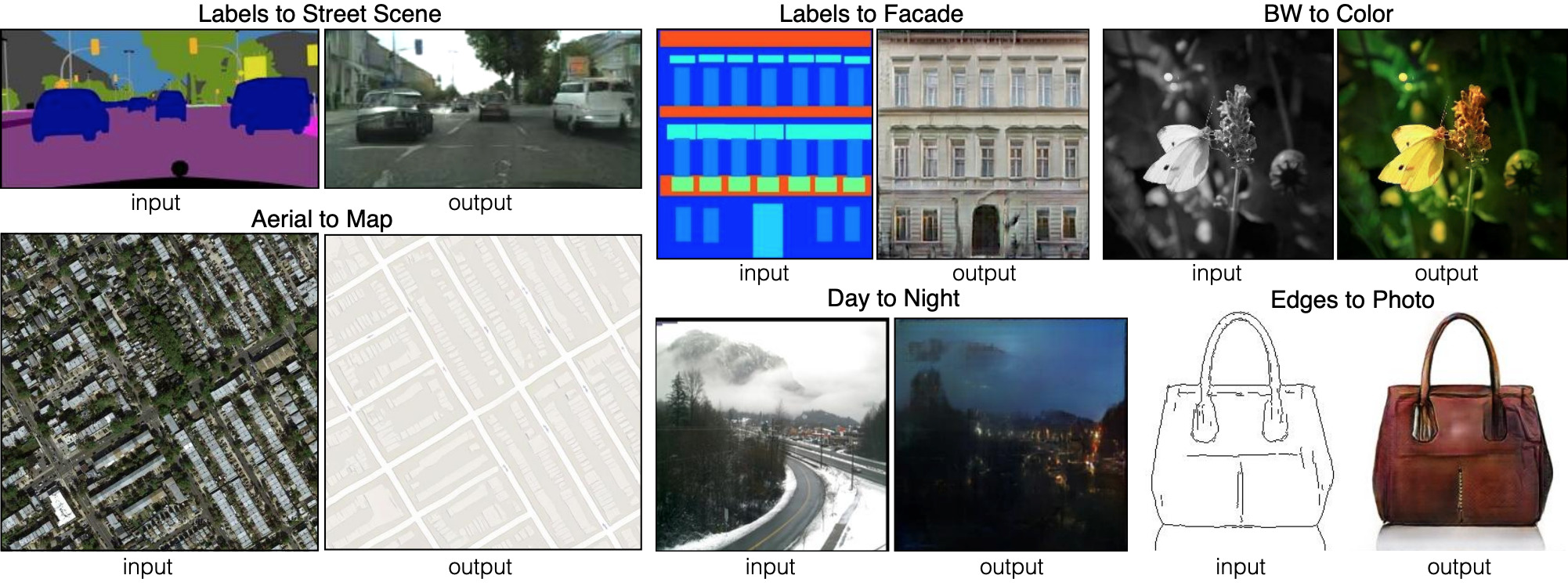Six different kinds of image-to-image translation, as demonstrated by pix2pix. pix2pix is a predecessor of the two networks we'll be looking at: pix2pixHD and SPADE. Source

For the generator to learn its mapping, it needs a dataset of segmentation maps and corresponding photos. We modify the GAN architecture so that the generator and discriminator both receive the segmentation map. The generator of course needs the map to know what it should draw where, but the discriminator also needs it to check that the generator put the correct kind of stuff in the right places.

The generator learns not to put grass where the segmentation map indicates “sky”, since otherwise the discriminator will easily determine the image to be fake.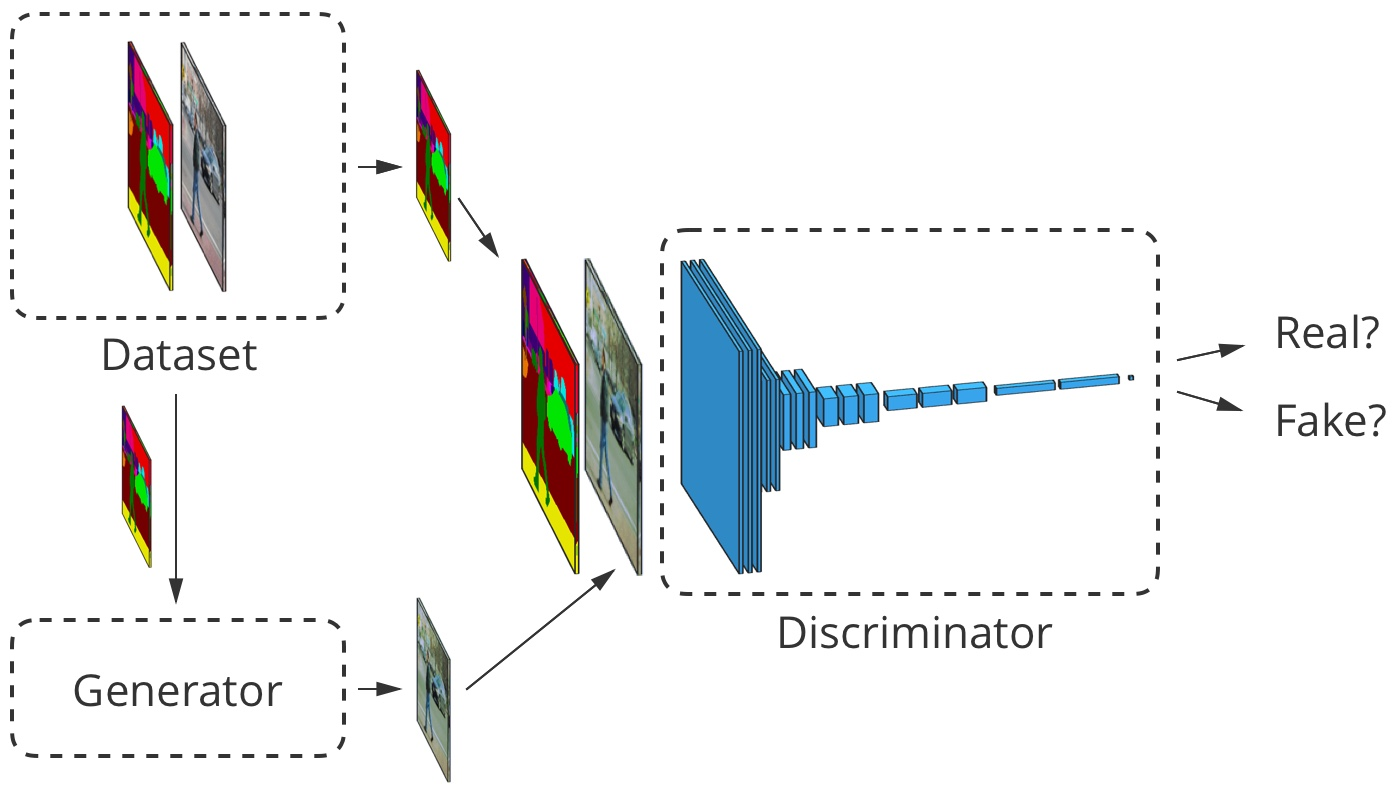For image-to-image translation, the input image is received by both the generator and the discriminator. The discriminator additionally receives either the generator output or the true output from the dataset. Example image from here

## Designing an image-to-image translator

Let’s look at a real image-to-image translator: pix2pixHD. SPADE derives much of its design from pix2pixHD.

For an image-to-image-translator, our generator both produces an image and takes one as input. We could simply have a stack of convolutional layers map between the two, but since convolutional layers only combine values in small patches, we’d need many layers to propagate information across a large image.

pix2pixHD solves this problem more efficiently by having a CNN that downscales the input image (the encoder) followed by one that upscales it to produce the output (the decoder). As we’ll soon see, SPADE has a different solution requiring no encoder.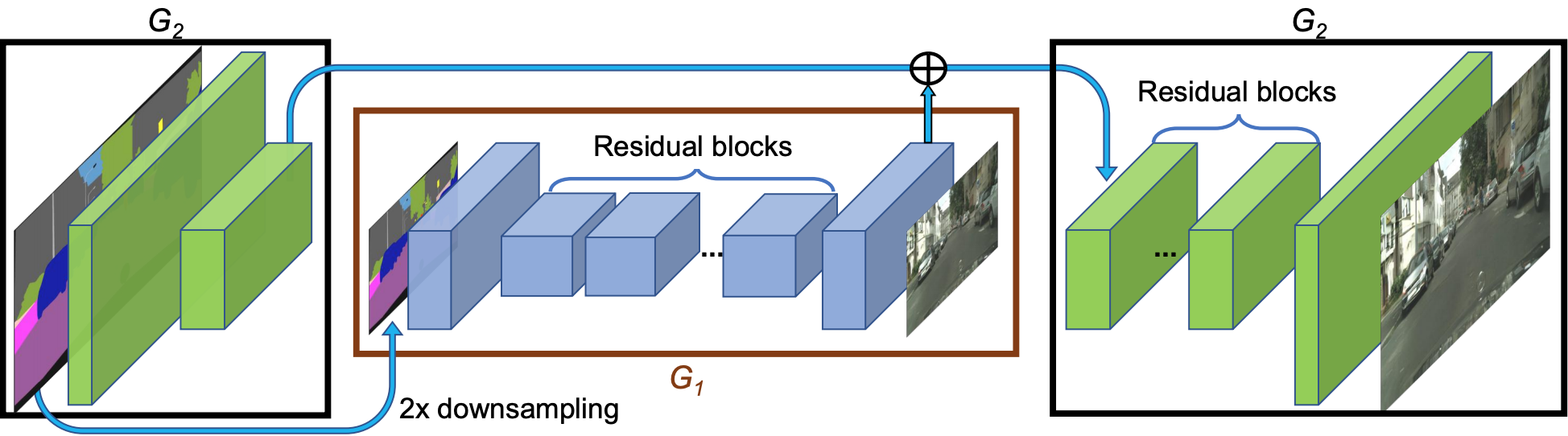The pix2pixHD network at a high level. The "residual" blocks and $+$ operation refer to skip connections. The network has skip connections between each scale in the encoder and the same scale in the decoder. Source

### Batch normalization is a challenge

Almost all modern convolutional neural networks use batch normalization or one of its siblings to significantly speed up and stabilize training. The activations of each channel have their mean moved to 0 and standard deviation to 1 before a pair of channel parameters $\beta$ and $\gamma$ allow them to “denormalize” again.

$$y = \frac{x - \mathrm{E}[x]}{\sqrt{\mathrm{Var}[x] + \epsilon}} * \gamma + \beta$$

Unfortunately, batch normalization hurts generators by making some types of image processing difficult for the network to implement. Instead of normalizing over a batch of images, pix2pixHD uses instance normalization which normalizes over each image separately.

### Training pix2pixHD

Modern GANs like pix2pixHD and SPADE measure the realism of their outputs a little differently than in the original design.

To deal with the challenges of generating high-resolution images, pix2pixHD uses three discriminators of identical structure, each of which receives the output image at a different scale (ordinary size, downscaled 2x and downscaled 4x).

For its loss function, pix2pixHD uses $V_\text{LSGAN}$, but also includes another term designed to help make the generator outputs more realistic (independent of whether it helps fool the discriminator). This term, $\mathcal{L}_\text{FM}$ (“feature matching”), encourages the generator to make the distribution of features (layer activations) in the discriminators similar between the real data and the generator outputs by minimizing the $L_1$ distance between them.

This gives an overall optimization goal of

$$\begin{equation*} \min_G \bigg(\lambda \sum_{k=1,2,3} V_\text{LSGAN}(G, D_k) + \big(\max_{D_1, D_2, D_3} \sum_{k=1,2,3} \mathcal{L}_\text{FM}(G, D_k)\big)\bigg) \end{equation*},$$

where the losses are summed over the 3 discriminators and the factor $\lambda = 10$ controls the relative importance of the two terms.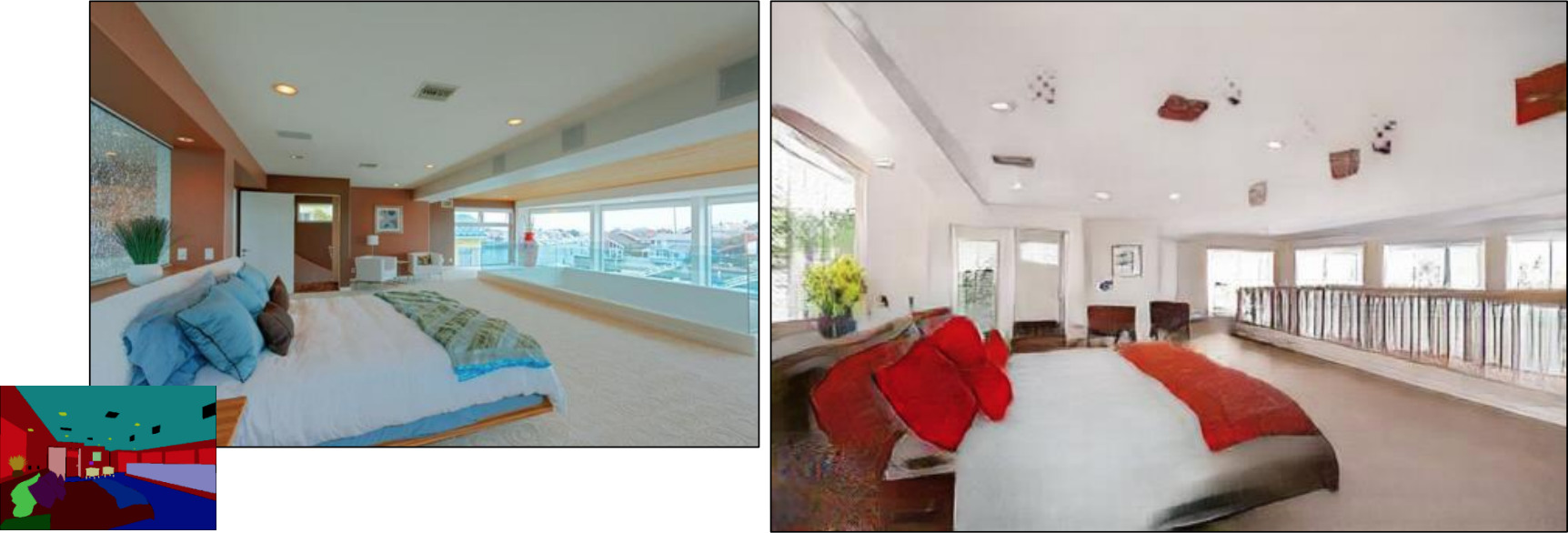pix2pixHD using a segmentation map drawn from a real bedroom (left in each example) to generate a fake bedroom (right). Modified from paper

While the discriminators could keep downscaling the image until they’re looking at image-wide features, they actually stop at only $70 \times 70$ patches (at their respective scales). Then they just sum all the values of those patches across the image.

This actually works well since the $\mathcal{L}_\text{FM}$ takes care of making the image look realistic at the large scale and $V_\text{LSGAN}$ is only needed to check the fine details. It also has the benefits of making the network faster, reducing the number of parameters it uses and allowing it to be used on any size of image.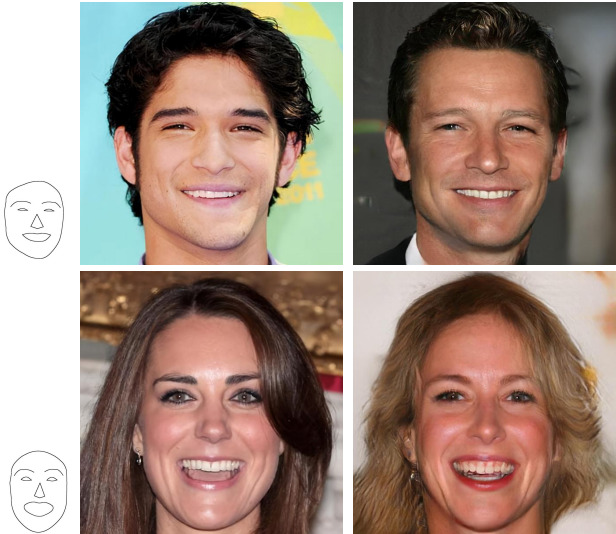pix2pixHD translating simple sketches of faces into photorealistic faces of matching expressions. Each example shows an image from the CelebA dataset in the middle, a sketch of that celebrity's facial expression to the left, and a face generated from the sketch to the right. Modified from paper

### What’s wrong with pix2pixHD?

Those results are incredible, but we can do better. It turns out pix2pixHD is falling short in an important way.

Consider what pix2pixHD does with a single-class input, say a map that puts grass everywhere. Because the input is spatially uniform, the outputs of the first convolutional layer are as well. Instance normalization then “normalizes” all the (identical) values for each channel in the image and produces $0$ as output for all of them. The $\beta$ parameter can shift this to a non-zero value, but the fact remains that the output will no longer depend on whether the input was “grass”, “sky”, or “water”.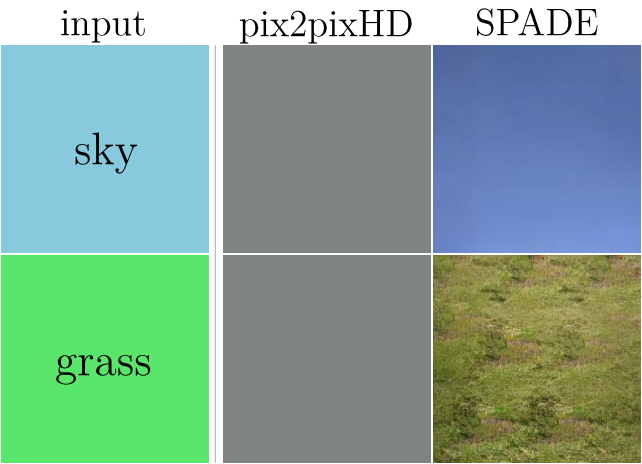In pix2pixHD, instance normalization tends to throw away information from the segmentation map. For single-class images, it produces the same image regardless of the class. Image from SPADE

Solving this problem is a central design criterion behind SPADE.

## The solution: SPADE

Finally we reach the new state-of-the-art for generating images from segmentation maps: spatially-adaptive (de)normalization (SPADE).

With SPADE the idea is to prevent the network from losing semantic information by allowing the segmentation map to control the normalization parameters $\gamma$ and $\beta$ locally in each layer. Rather than having just one pair of parameters for each channel, different pairs are computed for each spatial point by feeding a downsampled version of the segmentation map through 2 convolutional layers.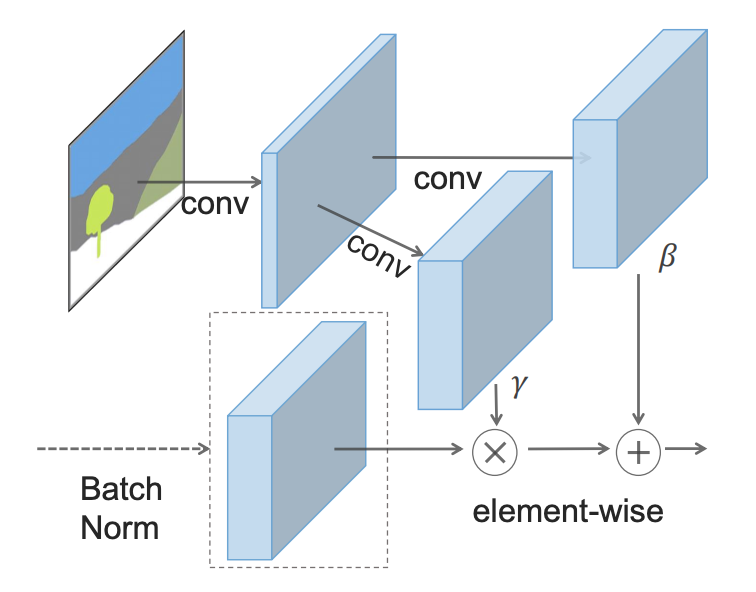Rather than input the segmentation map to the first layer, SPADE uses downsampled versions of it to modulate the batch-normalized outputs of every layer. Image from paper

The SPADE generator integrates this design into small “residual blocks” that get sandwiched between upsampling layers (transposed convolutions):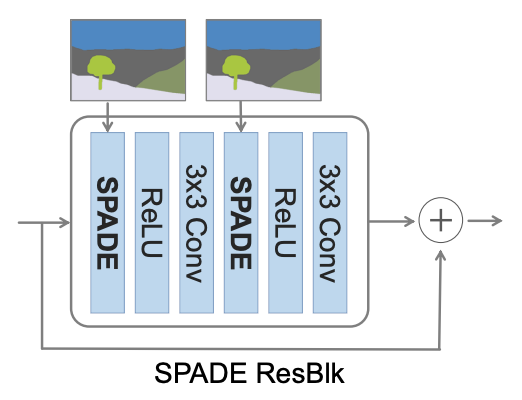SPADE's "residual blocks" include convolutional layers and skip connections. Source

Now that the segmentation map comes in “from the side” of the network, there’s no need to have it as the input to the first layer. Instead, we can go back to the original GAN design that used a random vector as input. That gives us the added bonus of being able to generate different images from a single segmentation map (“multimodal synthesis”). It also makes the whole “encoder” part of pix2pixHD unnecessary—a great simplification.

SPADE uses the same loss function as pix2pixHD with one minor change: instead of squaring the values in $V_\text{LSGAN}$ it uses the hinge loss.

With these changes, we get some great results: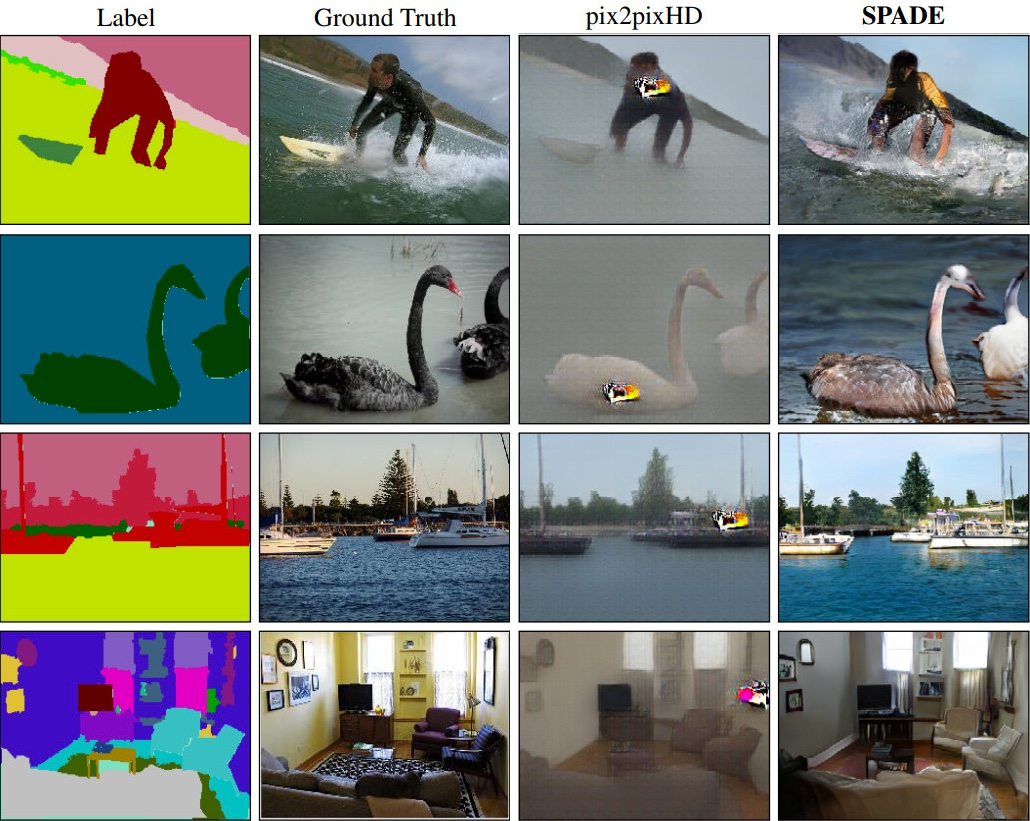SPADE compared with pix2pixHD on the COCO-stuff dataset. Modified from paper

### Intuition

Let’s think about how SPADE might produce the results it does. In the example below, we have a tree. GauGAN uses a single “tree” class to represent both the trunk and the leaves of the tree. Yet somehow it learns that the narrow part at the bottom of a “tree” is the trunk and should be brown, while the big blob above should be leafy.

The downsampled segmentation map that SPADE uses to modulate each layer may provide that hint.

You might notice that the trunk of the tree continues up into the large bushy part where even a 5x5 patch of the segmentation map would be entirely “tree”. So how does SPADE know to put more trunk there?

The answer is that the layer illustrated may receive information from lower resolution layers where a 5x5 block contains the entire tree. Each subsequent convolutional layer also allows some movement of information across the image.

The fact that SPADE allows the segmentation map to directly modulate each layer does not prevent the layers from also propagating related information onward as they do in pix2pixHD. It just prevents the semantic information from being lost, since it comes into each layer fresh.

### Style transfer

SPADE has one last piece of magic, which is the ability to generate an image in the style of a given image (e.g. lighting, weather patterns, season).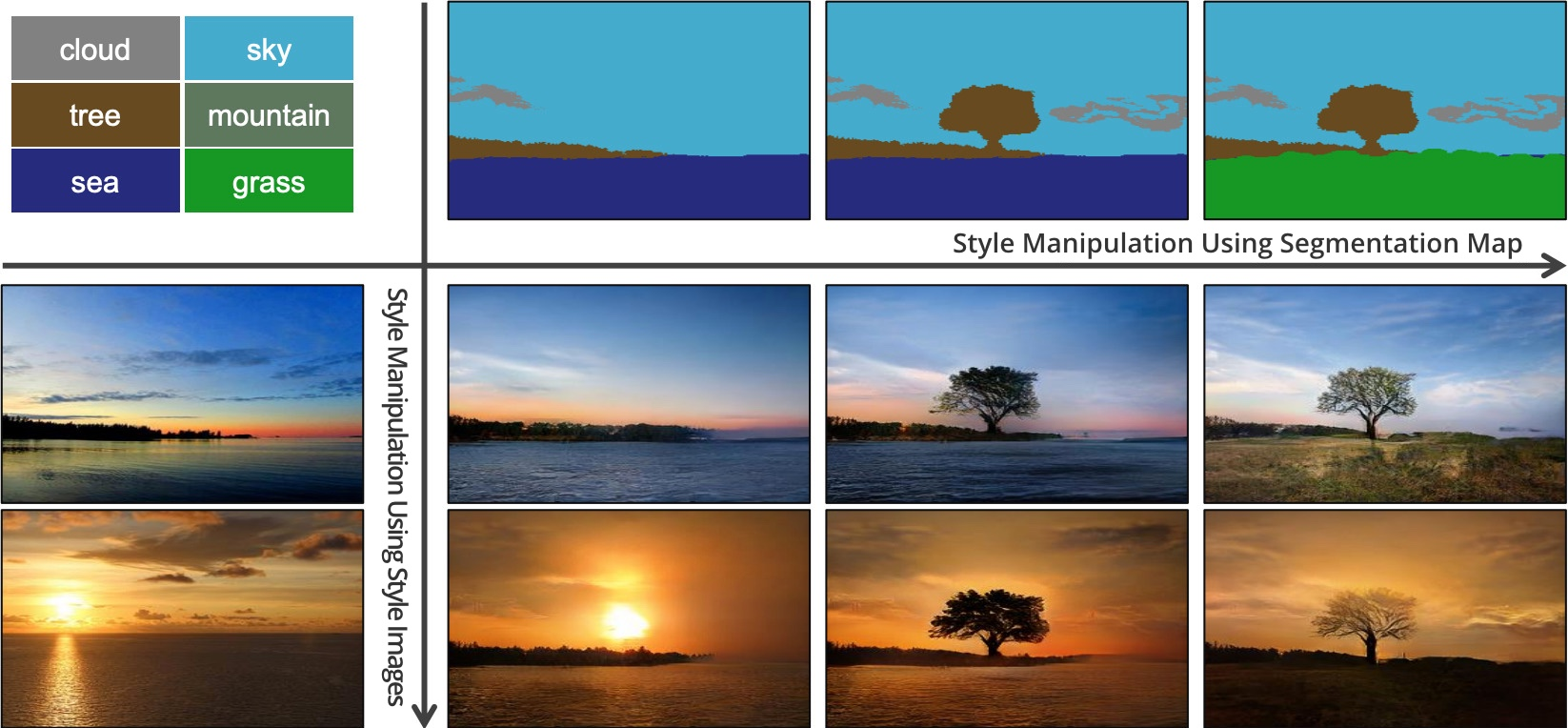SPADE can generate multiple styles of images from a single segmentation map by copying the style of a given image. Modified from paper

In short, this works by putting images through an encoder and training it to give the generator vectors $\vec{z}$ that will in turn reproduce similar images. Once the encoder is trained, we swap out the corresponding segmentation maps for arbitrary ones and SPADE’s generator makes images conforming to the new maps but in the style of the provided images.

Because the generator ordinarily expects samples from a multivariate normal distribution, we have to train the encoder to output values with a similar distribution if we want realistic outputs. This is the idea behind variational autoencoders, which Yoel Zeldes explains.

And that’s it for SPADE/GauGAN. I hope this exposition satisfied your curiosities about NVIDIA’s new system. For comments, business inquiries or anything else, message me on Twitter @AdamDanielKing or email adam@AdamDKing.com (note the D).